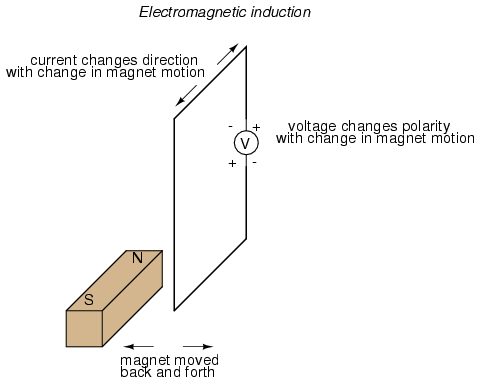• ×
السبت 6 يونيو 2020 | 05-28-2020
×

# القوة الدافعة الكهربية الحثية induced emfFaraday"s laws of of electromagnetic induction explains the relationship between electric circuit and magnetic field. This law is the basic working principle of the most of the electrical motors, generators, transformers, inductors etc.

Whenever a conductor is placed in a varying magnetic field an EMF gets induced across the conductor (called as induced emf), and if the conductor is a closed circuit then induced current flows through it.
Magnetic field can be varied by various methods -
1. By moving magnet
2. By moving the coil
3. By rotating the coil relative to magnetic field

Faraday"s second law of electromagnetic induction states that,  the magnitude of induced emf is equal to the rate of change of flux linkages with the coil. The flux linkages is the product of number of turns andthe flux associated with the coil.

Consider the conductor is moving in magnetic field, then
flux linkage with the coil at initial position of the conductor = NΦ1     (Wb) (N is speed of the motor and Φ is flux)
flux linkage with the coil at final position of the conductor = NΦ2       (Wb)
change in the flux linkage from initial to final = N(Φ1 - Φ2
let  Φ1 - Φ2 = Φ
therefore, change in the flux linkage = NΦ
and, rate of change in the flux linkage = NΦ/t
taking the derivative of RHS
rate of change of flux linkages = N (dΦ/dt)

According to Faraday"s law of electromagnetic induction, rate of change of flux linkages is equal to the induced emf

So, E = N (dΦ/dt)    (volts)

### Lenz"s Law

Lenz"s  law of electromagnetic induction states that, when an emf is induced according to Faraday"s law, the polarity (direction) of that induced emf is such that it opposes the cause of its production.

Thus, considering Lenz"s law

E = -N (dΦ/dt)   (volts)

The negative sign shows that, the direction of the induced emf and the direction of change in magnetic fields have opposite signs.

المصدر

بواسطة :
10-01-2016 12:00 صباحاً  0  0  2.5K
التعليقات ( 0 )